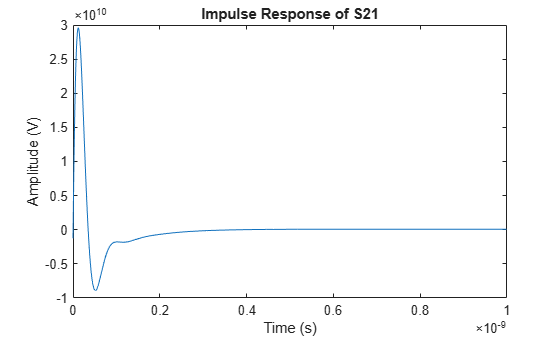# impulse

Impulse response for rational function object

## Syntax

```[resp,t] = impulse(h,ts,n) ```

## Description

`[resp,t] = impulse(h,ts,n)` computes the impulse response, `resp`, of the rational function object, `h`, over the time period specified by `ts` and `n`.

Note

While you can compute the output response for a rational function object by computing the impulse response of the object and then convolving that response with the input signal, this approach is not recommended. Instead, you should use the `timeresp` method to perform this computation because it generally gives a more accurate output signal for a given input signal.

The input `h` is the handle of a rational function object. `ts` is a positive scalar value that specifies the sample time of the computed impulse response, and `n` is a positive integer that specifies the total number of samples in the response.

The vector of time samples of the impulse response, `t`, is computed from the inputs as `t = [0,ts,2*ts,...,(n-1)*ts]`. The impulse response, `resp`, is an `n`-element vector of impulse response values corresponding to these times. It is computed using the analytical form of the rational function

`$resp=\sum _{k=1}^{M}{C}_{k}{e}^{{A}_{k}\left(t-Delay\right)}u\left(t-Delay\right)+D\delta \left(t-Delay\right)$`

where

• `A`, `C`, `D`, and `Delay` are properties of the rational function object, `h`.

• `M` is the number of poles in the rational function object.

## Examples

collapse all

Create a `sparameters` object from a file and use `rfparam` to extract the ${\mathit{S}}_{21}$parameters.

```S = sparameters('passive.s2p'); S21 = rfparam(S,2,1);```

Fit a rational function object to the ${\mathit{S}}_{21}$ data by using `rationalfit`.

```freq = S.Frequencies; fit_data = rationalfit(freq,S21)```
```fit_data = rfmodel.rational with properties: A: [6x1 double] C: [6x1 double] D: 0 Delay: 0 Name: 'Rational Function' ```

Calculate the impulse response using the `impulse` method and plot the results.

```[resp,t] = impulse(fit_data,1e-12,1e3); plot(t,resp);```